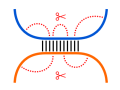Freiburg RNA Tools
Teaching - accessibility# Teaching - accessibility : of interacting sites source at github@BackofenLab/RNA-Playground

State-of-the-art RNA-RNA interaction prediction algorithms take the accessibility of the interacting regions into account. That is, a penalty corrects the interaction scoring for how much the interaction sites are involved in intramolecular base pairs. This can be expressed by reverting unpaired probabilities $P^{u}_{i,j}$ into pseudo energy scores via $-RT\log(P^{u}_{i,j})$ that represent the amount of energy (within the structure ensemble) to unfold a region $i..j$ in order to make it accessible for intermolecular RNA-RNA interactions (Ulrike Mückstein et al., 2006).

Here, we extend the hybrid-only approach towards the integration of an accessibility-based scoring. To this end, the simplified McCaskill approach is used to compute unpaired probabilities $P^{u}$. The penalties are used in a post-processing step to correct the hybridization energies in order to identify the interaction that optimizes the combination of hybridization and accessibility scoring.RNA sequence $S^1$:
RNA sequence $S^2$:
(Computation uses reversed sequence $\overleftarrow{S^2}$)
Minimal loop length $l$:
Energy weight of base pair $E_{bp}$:
'Normalized' temperature $RT$:
The following recursions are used to compute the interactions that show an optimal combination of hybridization and accessibility. To this end, first the maximal number of base pairs $D^{i,k}_{j,l}$ for all interaction sites are computed using the hybrid-only approach. Furthermore, the unpaired probabilities $P^{u1}$ and $P^{u2}$ are tabularized for both sequences $S^1$ and $\overleftarrow{S^2}$, resp., using the simplified McCaskill approach.
Given these values, the accessibility-incorporating interaction scorings are computed and stored in table $I$. A non-zero entry $I^{i,k}_{j,l}$ represents the combined scoring for an interaction of $S^1_{i..k}$ with $\overleftarrow{S^2_{j..l}}$ with left/right most base pairs $(S^1_i,\overleftarrow{S^2_j})$/$(S^1_k,\overleftarrow{S^2_l})$, respectively.

## Visualization of interacting base pairs (selected structure)

Due to the four-dimensionality of $I$, we only list the optimal hybrid structures (up to 15). On selection, the intermolecular base pairs are visualized. If all entries $I^{i,k}_{j,l}$ show negative scores, no favorable interaction is possible since intramolecular base pairs dominate the individual structures (too hight penalties for possible interaction sites). Therefore, the structure list might be empty.
Possible Structures
Energy of selection:
The box provides an ASCII representation of the interacting base pairs of the selected structure with $S^{1}$ on top and $S^{2}$ on the bottom. Note, sequence $S^{2}$ is reversed (running from right ($5'$) to left ($3'$)) within this representation. Note further, if no interacting base pairs are present, no visualization is done.

## Accessibility

The simplified McCaskill approach is used in order to compute the unpaired probabilities $P^{u}$ for each of the two sequences. Please refer to the according page for details. These are used to compute according energy penalties $ED$ to make an interacting site $i..j$ accessible, i.e. $ED_{i,j} = -RT\cdot\log(P^{u}_{i,j})$. In the following, the penalties for $S^1$ and $\overleftarrow{S^2}$ are visualized in dotplot format, i.e. the larger the dot the higher is the penalty for the respective subsequence to form an interaction.
Penalties $ED^{1}$ to make site in $S^{1}$ accessible:
Penalties $ED^{2}$ to make site in $\overleftarrow{S^2}$ accessible: